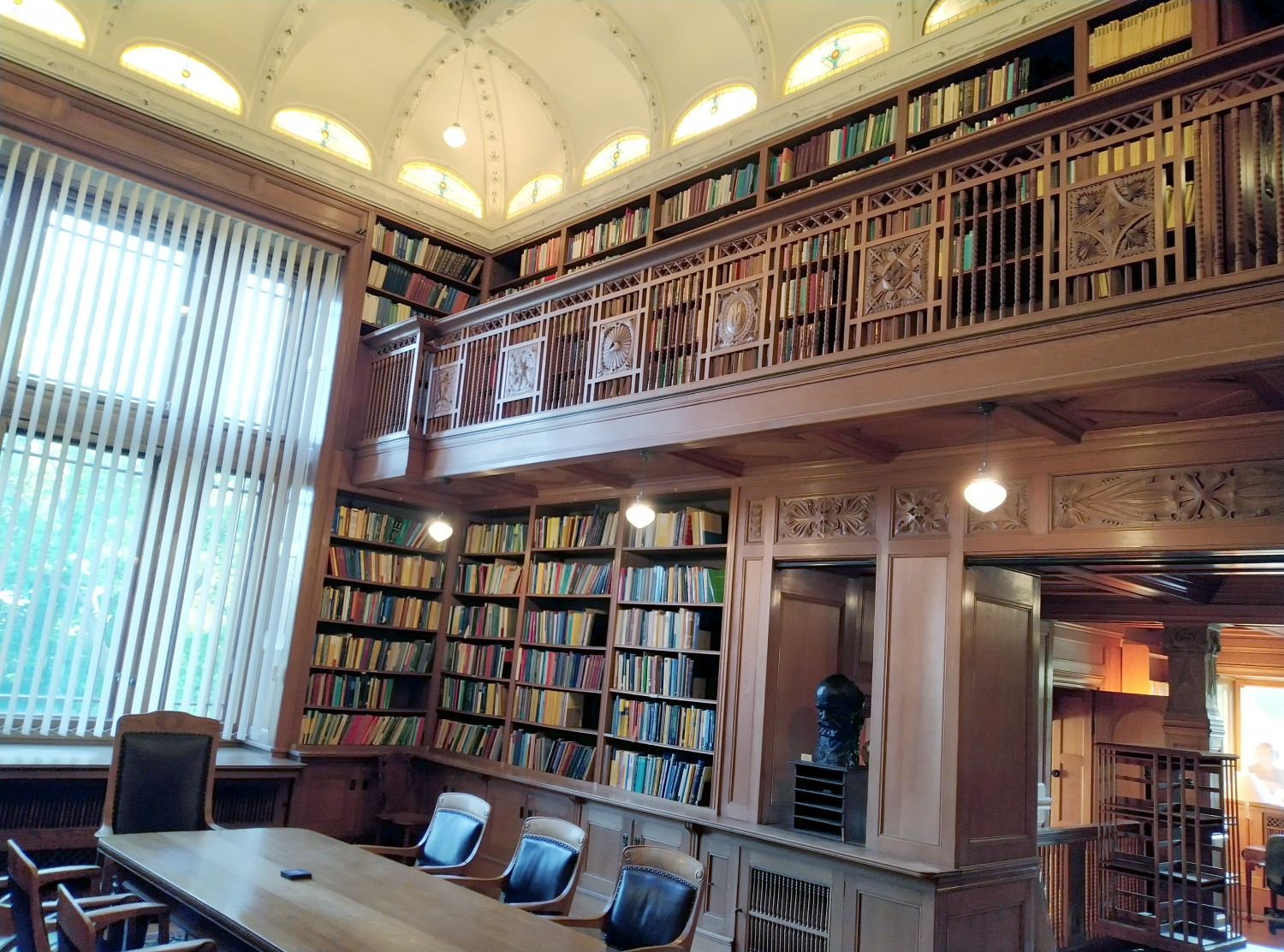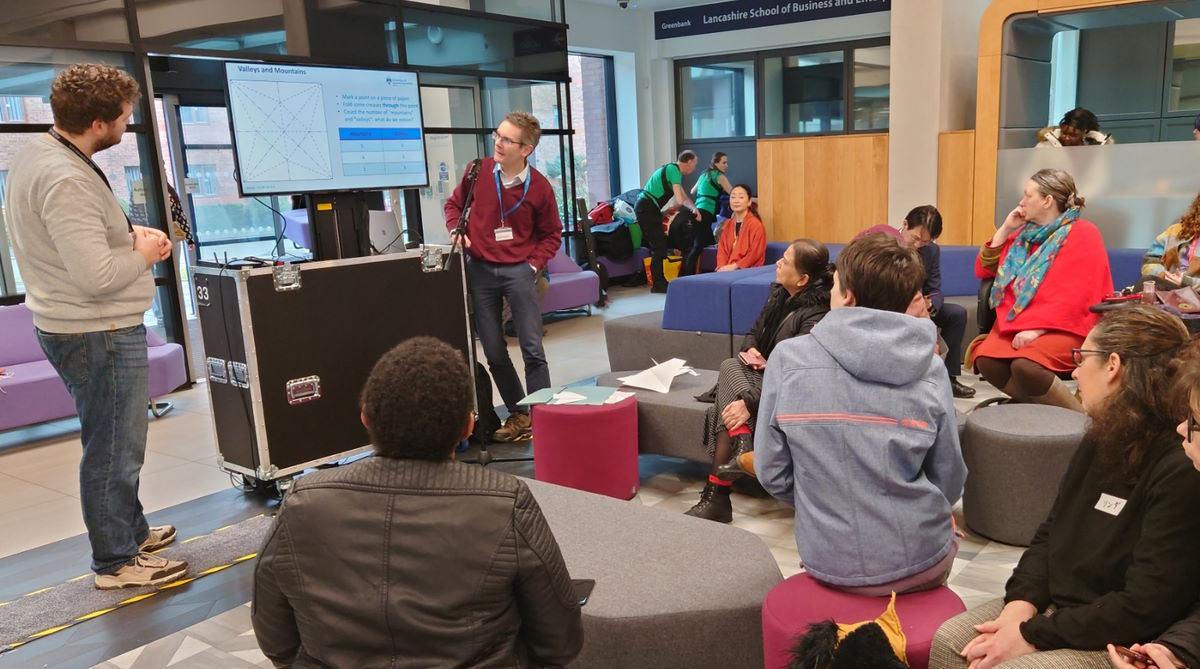# Blog of Matthew Daws

## Cheap analysis

This is a follow-on to an older post prompted by a comment from Yemon Choi (an old friend who is now a colleague). There are of course many examples of monotone Galois connections in Functional Analysis. Here is one example which I like.

Let $$E$$ be a Banach space. Let $$\mathcal X$$ be the collection of all linear subspaces of $$E$$, ordered by inclusion. Let $$\mathcal Y$$ be the collection of all linear subspaces of $$E^*$$ (the dual space of all continuous linear functionals) ordered by reverse inclusion.

Given $$F\in\mathcal X$$ we define $\mathcal L(F) = \{ f\in E^* : f(x)=0 \ (x\in F) \} \in \mathcal Y,$ the annihilator of $$F$$, easily seen to be a subspace. If $$F_1 \leq F_2$$ then $$F_1 \subseteq F_2$$ and so $$\mathcal L(F_1) \supseteq \mathcal L(F_2)$$ (if $$f$$ vanishes on $$F_2$$ it will vanish on $$F_1$$). So $$\mathcal L(F_1) \leq \mathcal L(F_2)$$ and $$\mathcal L$$ is order-preserving.

Similarly given $$F\in\mathcal Y$$ define $\mathcal R(F) = \{ x\in E : f(x)=0 \ (f\in F) \}\in \mathcal X,$ the pre-annihilator of $$F$$. This is again an order-preserving map.

## From pinchings to quantum colourings

Finally, I can combine the last post with a post 3 ago. This argument is from a paper of Elphick and Wocjan, attributed there to Roberson.

Fix a graph $$G$$ with adjacency matrix $$A$$.

Theorem: Let $$\mathcal C$$ be a pinching on $$\mathbb M_n\otimes\mathcal B(H)$$ with $$\mathcal C(A\otimes 1_H)=0$$ and $$\mathcal C(D\otimes 1_H)=D\otimes 1_H$$ for each diagonal matrix $$D$$. Then $$\mathcal C$$ arises from a quantum colouring of $$G$$, in the manner described before.

## Quantum chromatic numbers

Continuing from the last post I want to now consider the Quantum Chromatic Number of a graph. These ideas arose from Quantum Information Theory, which explore how quantum entanglement can be used in certain two-person "games", which are very similar to Interactive proof systems. While the motivation can be a little hard to explain, the resulting mathematical formalism is straightforward.

Definition: A quantum $$c$$-colouring of $$G$$ is a collection of orthogonal projections $$\{P_{v,k} : v\in V_G, k\in [c] \}$$ on some Hilbert space $$H$$ (usually assumed finite-dimensional) such that:

1. $$\sum_{k=1}^c P_{v,k} = 1_H$$ for each $$v$$;
2. $$P_{v,k} P_{w,k} = 0$$ for each $$k$$ and each edge $$v\sim w$$ in $$G$$.

There is a more complicated (but motivated) definition in [Cameron et al.] (see references below) where the reduction to this definition is essentially shown Proposition 1 and the later observations. It is also shown there that, when $$H$$ is finite dimensional, we can always assume that each projection has the same rank (though this is sometimes not natural.) The following is the same spectral bound which we discussed before, but now for the quantum chromatic number $$\chi_q(G)$$, the least $$c$$ for which $$G$$ has a quantum $$c$$-colouring.

Theorem: [Elphick-Wocjan] $$\chi_q(G) \geq 1+\frac{\lambda_1}{-\lambda_n}$$.

## Spectral bounds on the chromatic number

Recently Clive Elphink gave us a nice seminar where he discussed some of his "conjectures in spectral graph theory". Clive has an interesting history: after a career in business, he is now semi-retired and returned to research mathematics. By his own admission, he is more on the experimental side, and his talk said almost nothing about proofs. Here is a old result in this area:

Theorem [Hoffman]: We have that $$\chi(G) \geq 1 + \frac{\lambda_1}{-\lambda_n}$$.

I explain the notation shortly, but in words, this result relates a vertex colouring of a graph to the eigenvalues of the adjacency matrix. To me, this seems hugely surprising, as why would the spectrum of $$A$$ have anything to do with a vertex colouring? I want to explain the elegant arguments of Elphick and Wocjan, and also some generalisations to quantum colourings (in part 2).

## Fixed points of complete positive maps

The first of hopefully a few posts about actual Mathematics, the context of which will follow. In Watrous's QIT book, Theorem 4.25 states the following:

Let $$\Phi:\mathbb M_n\rightarrow\mathbb M_n$$ be a unital quantum channel with Kraus representation $$\Phi(x) = \sum_a A_a x A_a^*$$. Then $$\Phi(x)=x$$ if and only if $$A_a x = x A_a$$ for each $$a$$.

(Here I translate slightly the language). Whenever I see a statement about quantum channels, I ask myself if I can translate it into a more Operator Algebraic statement making more use of the Stinespring representation theorem, for example. After much messing about, I realised that the key property is that $$\Phi$$ is trace preserving, and that this, together with multiplicative domain theory, can be used to give a simple proof. (Well, maybe not simple, but one adding some context.)

## Cheap mathematics

A long-time-coming comment on a post by John Baez about "bargin-basement mathematics". (But let's not do ourselves down-- the rest of the system is more than happy enough to do that for us-- so I shall say "cheap" not quite "bargain-basement").

The setup here is a (monotone Galois connection), which consists of two posets $$X$$ and $$Y$$, and order-preserving maps $L:X\rightarrow Y, \qquad R:Y\rightarrow X,$ which satisfy $$L(x)\leq y$$ if and only if $$x\leq R(y)$$. The exercise, whose solution I'll give below, is that while $$L$$ and $$R$$ may of course fail to be mutual inverses, if we define $$X'=R(Y)$$ and $$Y'=L(X)$$ then $$L,R$$ restrict to $$X',Y'$$ respectively to become mutual inverses.

## Mittag-Leffler

I spend last week at the Institute Mittag-Leffler in a suburb of Stockholm, attending the Noncommutative Harmonic Analysis and Quantum Information conference. The institute is lovely, being comprised of a large, old mansion, various accommodation blocks, a seminar building, all set in lovely grounds. The weather couldn't have been nicer (well, too hot for me, but I'd probably be happy spending the summer near the arctic circle). As the history page explains, the mansion belonged to Mittag-Leffler, and was left in his will to form a Mathematics research institute, but sadly the Great Depression eroded his legacy to the extent that the institute couldn't function as envisaged until the latter part of the 20th century, when additional funding was found. If you get the chance to visit, I highly recommend it.

The mansion also houses an amazing Mathematics library. I took a little photo:## Origami

I got roped into (okay, volunteered for) giving a talk at UClan's Japan Day on the theme of Origami. To quote Tom Lehrer, this I know from nothing. But one can learn, and I hope I said something interesting in my short talk.